## GATE 2013

 Question 1

A binary operation ⊕ on a set of integers is defined as x ⊕ y = x+ y2. Which one of the following statements is TRUE about ⊕?

 A Commutative but not associative B Both commutative and associative C Associative but not commutative D Neither commutative nor associative
Engineering-Mathematics       Set-Theory
Question 1 Explanation:
Cumulative property:
A binary relation on a set S is called cumulative if a*b = b*a ∀ x,y∈S.
Associative property:
A binary relation on set is called associative if (a*b)*c = a*(b*c) ∀ x,y∈S.
Given x⊕y = x2 + y2 --------(1)
Replace x, y in (1)
y⊕x = y2 + x2 which is same as (1), so this is cumulative
(x⊕y)⊕z = (x2 + y2) ⊕ z
= (x2 + y2) + z2
= x2 + y2 + z2 + 2x2y2 ----------(2)
x⊕(y ⊕ z) = x ⊕ (y2 + z2)
= x2 + (y2 + z2)2
= x2 + y2 + z2 + 2y2z2 ----------- (3)
(2) & (3) are not same so this is not associative.
 Question 2

Suppose p is the number of cars per minute passing through a certain road junction between 5 PM and 6 PM, and p has a Poisson distribution with mean 3. What is the probability of observing fewer than 3 cars during any given minute in this interval?

 A 8/(2e3) B 9/(2e3) C 17/(2e3) D 26/(2e3)
Engineering-Mathematics       Probability
Question 2 Explanation:
The formula for the Poisson probability mean function
P(x : λ) = (e λx)/x! for x = 0,1,2….
‘λ’ is the average number (mean)
Given that mean = λ = 3
The probability of observing fewer than three cars is
P(zero car) + P(one car) + P(two cars)
= (e-3 30)/0!+(e-3 31)/1!+(e-3 32)/2!
= e-3+e-3∙3+(e-3)∙9)/2
= (17e-3<)/2
= 17/(2e3 )
 Question 3

Which one of the following does NOT equal toABCD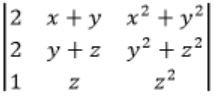Engineering-Mathematics       Linear-Algebra
Question 3 Explanation:Try to derive options from the given matrix.
Observe that col 2 + col 3 will reuse x(x+1) term
C2 → C1 + C2Question 4

The smallest integer that can be represented by an 8-bit number in 2’s complement form is

 A -256 B -128 C -127 D 0
Digital-Logic-Design       Number-Systems
Question 4 Explanation:
The range of 8-bit signed numbers representable is – 2n-1 to 2n-1 -1.
The smallest 8-bit 2’s complement number is 1000 0000.
MSB is 1. So it is a negative number.
To know the magnitude again take 2’s complement of 1000 0000.
1000 0000
0111 1111 ← 1’s complement
1000 0000 ← 2’s complement (1’s complement +1)
= 128
-128 is 1000 0000 in 2’s complement representation.
 Question 5

In the following truth table, V = 1 if and only if the input is valid.What function does the truth table represent?

 A Priority encoder B Decoder C Multiplexer D Demultiplexer
Digital-Logic-Design       Combinational-Circuits
Question 5 Explanation:
It is a 22 × 2 encoder. The inputs have priorities. So, it is a priority encoder.
 Question 6

Which one of the following is the tightest upper bound that represents the number of swaps required to sort n numbers using selection sort?

 A O(log n) B O(n) C O(n log n) D O(n2)
Algorithms       Sorting
Question 6 Explanation:
Best, Average and worst case will take maximum O(n) swaps.
Selection sort time complexity O(n2) in terms of number of comparisons. Each of these scans requires one swap for n-1 elements (the final element is already in place).
 Question 7

Which one of the following is the tightest upper bound that represents the time complexity of inserting an object into a binary search tree of n nodes?

 A O(1) B O(log n) C O(n) D O(n log n)
Algorithms       Sorting
Question 7 Explanation:
For skewed binary search tree on n nodes, the tightest upper bound to insert a node is O(n).
 Question 8

Consider the languages L1 = ϕ and L= {a}. Which one of the following represents L1L2* ∪ L1*?

 A {є} B ϕ C a* D {є,a}
Algorithms       Regular languages and Finite automata
Question 8 Explanation:
As we know, for any regular expression R,
Rϕ = ϕR = ϕ
So L1 L2 * = ϕ
and L1 * = {ϕ}* = {ϵ}
So L1L2* ∪ L1* = {ϵ}
 Question 9

What is the maximum number of reduce moves that can be taken by a bottom-up parser for a grammar with no epsilon- and unit-production (i.e., of type A → є and A → a) to parse a string with n tokens?

 A n/2 B n-1 C 2n-1 D 2n
Question 9 Explanation:
Since it is given that the grammar cannot have:
1) epsilon production
2) production of the form A → a
Consider the grammar:
S → Sa | a
If we were to derive the string “aaa” whose length is 3 then the number of reduce moves that would have been required are shown below:
S → Sa
→ Saa
→ aaa
This shows us that it has three reduce moves. The string length is 3 and the number of reduce moves is also 3. So presence of such kinds of production might give us the answer “n” for maximum number of reduce moves. But these productions are not allowed as per the question.
Also note that if a grammar does not have unit production then the maximum number of reduce moves can not exceed “n” where “n” denotes the length of the string.
3) No unit productions
Consider the grammar:
S → A
A → B
B → C
C → a
If we were to derive the string “a” whose length is 1 then the number of reduce moves that would have been required are shown below:
S → A
A → B
B → C
C → a
This shows us that it has four reduce moves. The string length is 1 and the number of reduce moves is 4. So presence of such kind of productions might give us the answer “n+1” or even more, for maximum number of reduce moves. But these productions are not allowed as per the question.
Now keeping in view the above points suppose we want to parse the string “abcd”. (n = 4) using bottom-up parsing where strings are parsed finding the rightmost derivation of a given string backwards. So here we are concentrating on deriving right most derivations only.
We can write the grammar which accepts this string which in accordance to the question, (i.e., with no epsilon- and unit-production (i.e., of type A → є and A → B) and no production of the form A → a) as follows:
S → aB
B → bC
C → cd
The Right Most Derivation for the above is:
S → aB (Reduction 3)
→ abC (Reduction 2)
→ abcd (Reduction 1)
We can see here the number of reductions present is 3.
We can get less number of reductions with some other grammar which also doesn’t produce unit or epsilon productions or production of the form A → a
S → abA
A → cd
The Right Most Derivation for the above is:
S → abA (Reduction 2)
→ abcd (Reduction 1)
Hence 2 reductions.
But we are interested in knowing the maximum number of reductions which comes from the 1st grammar. Hence total 3 reductions as maximum, which is (n – 1) as n = 4 here.
 Question 10

A scheduling algorithm assigns priority proportional to the waiting time of a process. Every process starts with priority zero (the lowest priority). The scheduler re-evaluates the process priorities every T time units and decides the next process to schedule. Which one of the following is TRUE if the processes have no I/O operations and all arrive at time zero?

 A This algorithm is equivalent to the first-come-first-serve algorithm. B This algorithm is equivalent to the round-robin algorithm. C This algorithm is equivalent to the shortest-job-first algorithm. D This algorithm is equivalent to the shortest-remaining-time-first algorithm.
Operating-Systems       CPU-Scheduling
Question 10 Explanation:
The given algorithm is equivalent to the round robin algorithm with time quantum T units.
 Question 11

Match the problem domains in GROUP I with the solution technologies in GROUP II

```       GROUP I                                       GROUP II
(P) Service oriented computing                 (1) Interoperability
(Q) Heterogeneous communicating systems        (2) BPMN
(R) Information representation                 (3) Publish-find-bind
(S) Process description                        (4) XML```
 A P-1, Q-2, R-3, S-4 B P-3, Q-4, R-2, S-1 C P-3, Q-1, R-4, S-2 D P-4, Q-3, R-2, S-1
Software-Engineering       SE
Question 11 Explanation:
Note: Out of syllabus [Web Technologies].
 Question 12

The transport layer protocols used for real time multimedia, file transfer, DNS and email, respectively are

 A TCP, UDP, UDP and TCP B UDP, TCP, TCP and UDP C UDP, TCP, UDP and TCP D TCP, UDP, TCP and UDP
Computer-Networks       Transport Layer
Question 12 Explanation:
Real time multimedia needs connectionless service, so underlying transport layer protocol used is UDP. File transfer runs over TCP protocol with port no-21.
DNS runs over UDP protocol within port no-53.
Email needs, SMTP protocol which runs over TCP protocol within port no –25.
 Question 13

Using public key cryptography, X adds a digital signature  to message M, encrypts <M, σ>, and sends it to Y, where it is decrypted. Which one of the following sequences of keys is used for the operations?

 A Encryption: X’s private key followed by Y’s private key; Decryption: X’s public key followed by Y’s public key B Encryption: X’s private key followed by Y’s public key; Decryption: X’s public key followed by Y’s private key C Encryption: X’s public key followed by Y’s private key; Decryption: Y’s public key followed by X’s private key D Encryption: X’s private key followed by Y’s public key; Decryption: Y’s private key followed by X’s public key
Computer-Networks       Network-Security
Question 13 Explanation:Encryption: Source has to encrypt with its private key for forming Digital signature for Authentication. Source has to encrypt the (M, σ) with Y’s public key to send it confidentially.
Decryption: Destination Y has to decrypt first with its private key, then decrypt using source public key.
 Question 14

Assume that source S and destination D are connected through two intermediate routers labeled R. Determine how many times each packet has to visit the network layer and the data link layer during a transmission from S to D.A Network layer – 4 times and Data link layer – 4 times B Network layer – 4 times and Data link layer – 3 times C Network layer – 4 times and Data link layer – 6 times D Network layer – 2 times and Data link layer – 6 times
Computer-Networks       Network-Layer
Question 14 Explanation:From above given diagram, its early visible that packet will visit network layer 4 times, once at each node [S, R, R, D] and packet will visit Data Link layer 6 times. One time at S and one time at D, then two times for each intermediate router R as data link layer is used for link to link communication.
One at packet reaches R and goes up from physical –DL-Network and second time when packet coming out of router in order Network –DL-Physical.
 Question 15

An index is clustered, if

 A it is on a set of fields that form a candidate key. B it is on a set of fields that include the primary key. C the data records of the file are organized in the same order as the data entries of the index. D the data records of the file are organized not in the same order as the data entries of the index.
Database-Management-System       B+-Trees
Question 15 Explanation:
Option (A) and Option (B) are not correct because, index can be created using any column or combination of columns, which need not be unique.
Basically, Indexed column is used to sort the rows of table. Whole data record of file is sorted using index so the correct option is (C).
 Question 16

Three concurrent processes X, Y, and Z execute three different code segments that access and update certain shared variables. Process X executes the P operation (i.e., wait) on semaphores a, b and c; process Y executes the P operation on semaphores b, c and d; process Z executes the P operation on semaphores c, d, and a before entering the respective code segments. After completing the execution of its code segment, each process invokes the V operation (i.e., signal) on its three semaphores. All semaphores are binary semaphores initialized to one. Which one of the following represents a deadlock-free order of invoking the P operations by the processes?

 A X: P(a)P(b)P(c) Y: P(b)P(c)P(d) Z: P(c)P(d)P(a) B X: P(b)P(a)P(c) Y: P(b)P(c)P(d) Z: P(a)P(c)P(d) C X: P(b)P(a)P(c) Y: P(c)P(b)P(d) Z: P(a)P(c)P(d) D X: P(a)P(b)P(c) Y: P(c)P(b)P(d) Z: P(c)P(d)P(a)
Operating-Systems       Process-Management
Question 16 Explanation:
Trick to check deadlock in the given code proceed parallely i.e., first executed the first line of all the processes then executed the second line of all processes and so on.
 Question 17

Which of the following statements is/are FALSE?

1. For every non-deterministic Turing machine, there exists an equivalent deterministic Turing machine.
2. Turing recognizable languages are closed under union and complementation.
3. Turing decidable languages are closed under intersection and complementation.
4. Turing recognizable languages are closed under union and intersection.
 A 1 and 4 only B 1 and 3 only C 2 only D 3 only
Theory-of-Computation       REL&Turing-Machines
Question 17 Explanation:
As we can convert a non-deterministic TM into an equivalent deterministic Turing machine so the statement “for every non-deterministic Turing machine, there exists an equivalent deterministic Turing machine” is true.
Turing recognizable means recursively enumerable languages which is closed under UNION but they are not closed under complementation, so statement 2 is false.
Turing decidable means recursive languages and they are closed under Intersection and complementation.
Turing recognizable means recursively enumerable languages which is closed under UNION and INTERSECTION.
 Question 18

Which of the following statements are TRUE?

1. The problem of determining whether there exists a cycle in an undirected graph is in P.
2. The problem of determining whether there exists a cycle in an undirected graph is in NP.
3. If a problem A is NP-Complete, there exists a non-deterministic polynomial time algorithm to solve A.
 A 1, 2 and 3 B 1 and 2 only C 2 and 3 only D 1 and 3 only
Theory-of-Computation       NP-Complete
Question 18 Explanation:
Note: Out of syllabus.
1. Detecting cycle in a graph using DFS takes O(V+E) = O(V2)
Here, for complete graph E <= V2. So, It runs in polynomial time.
2. Every P-problem is NP because P subset of NP (P ⊂ NP)
3. NP – complete ∈ NP.
Hence, NP-complete can be solved in non-deterministic polynomial time.
 Question 19

What is the time complexity of Bellman-Ford single-source shortest path algorithm on a complete graph of n vertices?

 A Θ(n2) B Θ(n2 log n) C Θ(n3) D Θ(n3 log n)
Algorithms       Sorting
Question 19 Explanation:
The Bellman–Ford algorithm is an algorithm that computes shortest paths from a single source vertex to all of the other vertices in a weighted digraph. It is capable of handling graphs in which some of the edge weights are negative numbers.
Bellman–Ford runs in O(|V| * |E|) time, where |V| and |E| are the number of vertices and edges respectively.
Note: For complete graph: |E| = n(n-1)/2 = O(n2)
 Question 20

In a k-way set associative cache, the cache is divided into v sets, each of which consists of k lines. The lines of a set are placed in sequence one after another. The lines in set s are sequenced before the lines in set (s+1). The main memory blocks are numbered 0 onwards. The main memory block numbered j must be mapped to any one of the cache lines from

 A (j mod v) * k to (j mod v) * k + (k-1) B (j mod v) to (j mod v) + (k-1) C (j mod k) to (j mod k) + (v-1) D (j mod k) * v to (j mod k) * v + (v-1)
Computer-Organization       Cache
Question 20 Explanation:
Number of sets in cache = v.
A block numbered j will be mapped to set number (j mod v). Since it is k-way set associative cache, there are k blocks in each set. It is given in the question that the blocks in consecutive sets are sequenced. It means for set-0 the cache lines are numbered 0, 1, .., k-1 and for set-1, the cache lines are numbered k, k+1,... k+k-1 and so on. As the main memory block j will be mapped to set (j mod v), it will be any one of the cache lines from (j mod v) * k to (j mod v) * k + (k-1).
 Question 21

Which one of the following expressions does NOT represent exclusive NOR of x and y?

 A xy+x'y' B x⊕y' C x'⊕y D x'⊕y'
Digital-Logic-Design       Number-Systems
Question 21 Explanation:
x ⊕ y = x’y + xy’
x’ ⊕ y’ = xy’ + x’y = x⊕y. Hence option D is correct.
 Question 22

Which one of the following functions is continuous at x = 3?

 ABC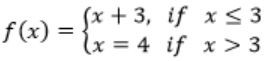D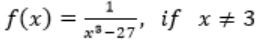Engineering-Mathematics       Number-System-and-Calculus
Question 22 Explanation:
Option A:
At x = 3, f(x) = 2
LHL (3), f(3-) = (x+3 )/3 = (3+3)/3 = 2
RHL (3), f(3+) = x-1 = 3-1 = 2
∴ f(x) is continuous.
Option B:
At x = 3, f(x) = 4
For x ≠ 3, f(x) = f(3+) = f(3-) = 8-x = 8-3 = 5
This is not continuous.
Option C:
At x ≤ 3, f(x) = x+3 = 3+3 = 6
At RHL(3), f(3+) = 4
This is not continuous.
Option D:
f(x) at x = 3 is not defined.
There is a break at x = 3, so this is not continuous.
 Question 23

Function f is known at the following points: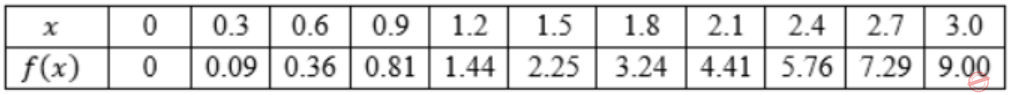The value of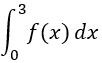computed using the trapezoidal rule is

 A 8.983 B 9.003 C 9.017 D 9.045
Engineering-Mathematics       Number-System-and-Calculus
Question 23 Explanation:
Note: Numerical methods are not in GATE syllabus now.
 Question 24

Consider an undirected random graph of eight vertices. The probability that there is an edge between a pair of vertices is 1/2. What is the expected number of unordered cycles of length three?

 A 1/8 B 1 C 7 D 8
Algorithms       Graph-Theory
Question 24 Explanation:
Among available ‘8’ vertices, we need to identify the cycles of length ‘3’.The probability that there exists one edge between two vertices = 1/2So, the total probability that all three edges of the above exists
= 1/2 × 1/2 × 1/2 (as they are independent events)
= 1/8
Total number of ways in which we can select ‘3’ such vertices among ‘8’ vertices = 8C3 = 56
Total number of cycles of length ‘3’ out of 8 vertices = 56 × 1/8 = 7
 Question 25

Which of the following statements is/are TRUE for undirected graphs?

P: Number of odd degree vertices is even.
Q: Sum of degrees of all vertices is even.
 A P only B Q only C Both P and Q D Neither P nor Q
Algorithms       Graph-Theory
Question 25 Explanation:
Euler’s Theorem 3:
The sum of the degrees of all the vertices of a graph is an even number (exactly twice the number of edges).
In every graph, the number of vertices of odd degree must be even.
 Question 26

P only
P and R only
R only
P, Q and S only
Question 26 Explanation:
P) True. Because every edge in cycle graph will become a vertex in new graph L(G) and every vertex of cycle graph will become an edge in new graph.
R) False. We can give counter example. Let G has 5 vertices and 9 edges which is planar graph. Assume degree of one vertex is 2 and of all others are 4. Now, L(G) has 9 vertices (because G has 9 edges) and 25 edges. But for a graph to be planar,
|E| ≤ 3|v| - 6
For 9 vertices |E| ≤ 3 × 9 - 6
⇒ |E| ≤ 27 - 6
⇒ |E| ≤ 21. But L(G) has 25 edges and so is not planar.
As (R) is false, option (B) & (C) are eliminated.
 Question 27

The line graph L(G) of a simple graph G is defined as follows: · There is exactly one vertex v(e) in L(G) for each edge e in G. · For any two edges e and e' in G, L(G) has an edge between v(e) and v(e'), if and only if e and e'are incident with the same vertex in G. Which of the following statements is/are TRUE?

(P) The line graph of a cycle is a cycle.
(Q) The line graph of a clique is a clique.
(R) The line graph of a planar graph is planar.
(S) The line graph of a tree is a tree.
 A P only B P and R only C R only D P, Q and S only
Algorithms       Graph-Theory
Question 27 Explanation:
P) True. Because every edge in cycle graph will become a vertex in new graph L(G) and every vertex of cycle graph will become an edge in new graph.
R) False. We can give counter example. Let G has 5 vertices and 9 edges which is planar graph. Assume degree of one vertex is 2 and of all others are 4. Now, L(G) has 9 vertices (because G has 9 edges) and 25 edges. But for a graph to be planar,
|E| ≤ 3|v| - 6
For 9 vertices |E| ≤ 3 × 9 - 6
⇒ |E| ≤ 27 - 6
⇒ |E| ≤ 21. But L(G) has 25 edges and so is not planar.
As (R) is false, option (B) & (C) are eliminated.
 Question 28

What is the logical translation of the following statement?

`  "None of my friends are perfect."`
 A ∃x(F(x)∧¬P(x)) B ∃x(¬F(x)∧P(x)) C ∃x(¬F(x)∧¬P(x)) D ¬∃x(F(x)∧P(x))
Engineering-Mathematics       Prepositional-Logic
Question 28 Explanation:
Let F(x) = x is my friend
P(x) = x is perfect
The meaning of ∃x(P(x)∧F(x)) is atleast one person who is my friend and perfect.
The negation of ∃x(P(x)∧F(x)) is “This is not the case that atlease one person who is my friend and perfect”.
So ~∃x(P(x)∧F(x)) is none of my friends are perfect.
 Question 29

Consider the following sequence of micro-operations.

```     MBR ← PC
MAR ← X
PC ← Y
Memory ← MBR```

Which one of the following is a possible operation performed by this sequence?

 A Instruction fetch B Operand fetch C Conditional branch D Initiation of interrupt service
Computer-Organization       CPU-Control-Design-and-Interfaces
Question 29 Explanation:
We know PC holds the value of the next instruction to be executed. Here the value of PC is stored in MBR( MBR ← PC ) and then to the memory (Memory ← MBR). We are saving the value of PC in memory and a new address is loaded into the PC (PC ← Y), so we start executing from the new address Y which is loaded into PC. So this behaviour matches with the interrupt service execution as we have already saved the address of current running instruction into memory and then we are loading new address in to the PC and start executing from there. Hence option D is the answer.
 Question 30

Consider a hard disk with 16 recording surfaces (0-15) having 16384 cylinders (0-16383) and each cylinder contains 64 sectors (0-63). Data storage capacity in each sector is 512 bytes. Data are organized cylinder-wise and the addressing format is <cylinder no., surface no., sector no.>. A file of size 42797 KB is stored in the disk and the starting disk location of the file is <1200, 9, 40>. What is the cylinder number of the last sector of the file, if it is stored in a contiguous manner?

 A 1281 B 1282 C 1283 D 1284
Operating-Systems       Input-and-output-system
Question 30 Explanation:
It is given that we have 16 recording surfaces and 16384 cylinders, each cylinder contains 64 sectors and starting address is <1200, 9, 40>. Capacity of each sector is 512Bytes.
Suppose we have x cylinders & in each cylinder we have 16 surface and 64 sectors so we need to store 42797 KB of data.
x * 16* 64 *512 + 16*64* 512 + 64* 512 = 42797 KB , by solving this we get x = 82.5 so we need 83 cylinders.
We can add this to the no. of cylinders in the starting address <1200, 9 ,40 >, i.e. 1200, but we also need to cover 40 more sectors which will need one more cylinder, this cylinder is not full but still it has to be accommodated.
Hence 1200+83+1 = 1284 and currently we will be on 1284 cylinder.
 Question 31

The number of elements that can be sorted in Θ(log n) time using heap sort is

 A Θ(1) B Θ(√log⁡n) C Θ (log⁡n/log⁡log⁡n) D Θ(log n)
Algorithms       Sorting
Question 31 Explanation:
Using heap sort to n elements it will take O(n log n) time. Assume there are log n/ log log n elements in the heap.
So, Θ((logn/ log log n)log(logn/log log n))
= Θ(logn/log logn (log logn - log log logn))
= Θ((log n/log logn) × log log n)
= Θ(log n)
Hence, option (C) is correct answer.
 Question 32

Consider the following function:

```int unknown(int n) {
int i, j, k = 0;
for (i  = n/2; i <= n; i++)
for (j = 2; j <= n; j = j * 2)
k = k + n/2;
return k;
}
```
 A Θ(n2) B Θ(n2 log n) C Θ(n3) D Θ(n3 logn)
Programming       Programming
Question 32 Explanation:
Outer loop runs for (n/2) times and inner loop runs for (logn) times.
So, the total number of times loop runs is (n/2 logn).
So, the final k value will be n/2*(n/2 logn) = O(n2logn)
= (n/2+1).n/2 ∙log n
= (n2log n)
 Question 33

Consider the following languages.

L1 = {0p1q0r|p,q,r≥0}
L2 = {0p1q0r|p,q,r≥0, p≠r}

Which one of the following statements is FALSE?

 A L2 is context-free. B L1∩ L2 is context-free. C Complement of L2 is recursive. D Complement of L1 is context-free but not regular.
Programming       CFL&PDA
Question 33 Explanation:
L1 = 0*1*0* which is regular language and in L2 we have one comparison (i.e. p ≠ r) so it is CFL.
Intersection of a regular language with a context free language is context free language (by closure properties of regular language) So L1∩ L2 is context-free.
L2 is CFL and CFL is not closed under complementation so we have to assume L2 as CSL (since every CFL is CSL) and CSL is closed under complement so Complement of L2 must be CSL and every CSL is recursive so Complement of L2 is recursive.
Since L1 is regular and regular language is closed under complement so complement of L1 must be regular and hence the statement Complement of L1 is context-free but not regular is a false statement.
 Question 34

Consider the DFA given.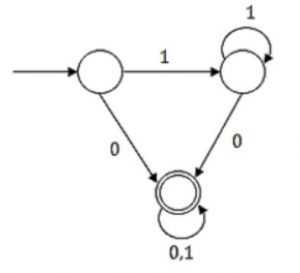Which of the following are FALSE?

Which of the following are FALSE?

1. Complement of L(A) is context-free.
2. L(A) = L((11*0+0)(0 + 1)*0*1*)
3. For the language accepted by A, A is the minimal DFA.
4. A accepts all strings over {0, 1} of length at least 2.
 A 1 and 3 only B 2 and 4 only C 2 and 3 only D 3 and 4 only
Theory-of-Computation       Regular Languages and Finite Automata
Question 34 Explanation:
L(A) is regular and its complement is also regular (by closure property) and every regular is CFL also. So Complement of LA is context-free.
The regular expression corresponding to the given FA isHence we have regular expression: (11*0 +0) (0+1)*
Since we have (0+1)* at the end so if we write 0*1* after this it will not have any effect, the reason is whenever string ends with the terminals other than 1*0* there we can assume 1*0* as epsilon.
So it is equivalent to (11*0 +0) (0+1)*0*1*
The given DFA can be minimised, since the non-final states are equivalent and can be merged and the min DFA will have two states which is given below:Hence statement 3 is false.
Since DFA accept string “0” whose length is one, so the statement “A accepts all strings over {0, 1} of length at least 2” is false statement.
 Question 35

A shared variable x, initialized to zero, is operated on by four concurrent processes W, X, Y, Z as follows. Each of the processes W and X reads x from memory, increments by one, stores it to memory, and then terminates. Each of the processes Y and Z reads x from memory, decrements by two, stores it to memory, and then terminates. Each process before reading x invokes the P operation (i.e., wait) on a counting semaphore S and invokes the V operation (i.e., signal) on the semaphore S after storing x to memory. Semaphore S is initialized to two. What is the maximum possible value of x after all processes complete execution?

 A -2 B -1 C 1 D 2
Operating-Systems       Process-Management
Question 35 Explanation: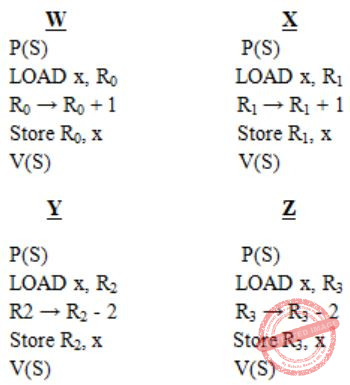Suppose, 'W' executes first, and makes value of semaphore S=1. Now it increment value of x to 1 and comes out and make S=2. Now 'X' executes, where the value of x=1 is stored in R1 and then R1 is incremented and value in R1 is 2. Now 'X' gets preempted. Also to be noted that value of semaphore S is now '1' because the execution during process X got preempted and wasn't able to increase value of S to 2.
Now process Y will get completely execute and then Z will get completely execute. Now the value of 'x' is -3. Now again 'X' will get executed and the value stored in R1 i.e., '2' will get stored in 'x'. So the value in 'x' will be finally 2.
So the maximum possible value of 'x' is 2.
 Question 36

Consider the following relation schema

Students(rollno: integer, sname: string)
Courses(courseno: integer, cname: string)
Registration(rollno: integer, courseno: integer, percent: real)

Which of the following queries are equivalent to this query in English?

`"Find the distinct names of all students who score more than 90% in the course numbered 107" `
```(I) SELECT DISTINCT S.sname
FROM Students as S,Registration as R
WHERE R.rollno = S.roll AND R.courseno = 107 AND R.percent > 90
(II) Πsname(σcourseno=107∧percent>90(Registration ⋈ Students))
(III) {T|∃S ∈ Students, ∃R ∈ Registration (S.rollno = R.rollno ∧ R.courseno = 107 ∧ R.percent > 90 ∧ T.sname = S.sname)}
(IV) {〈SN〉|∃SR∃RP(〈SR,SN〉 ∈ Students ∧ 〈SR,107,RP〉 ∈ Registration ∧ RP>90)}
```
 A I, II, III and IV B I, II and III only C I, II and IV only D II, III and IV only
Database-Management-System       ER-Model
Question 36 Explanation:
Four queries given in SQL, RA, TRC and DRC and all four statements will retrieve the required information.
 Question 37

Determine the maximum length of the cable (in km) for transmitting data at a rate of 500 Mbps in an Ethernet LAN with frames of size 10,000 bits. Assume the signal speed in the cable to be 2,00,000 km/s.

 A 1 B 2 C 2.5 D 5
Question 37 Explanation:
500×106 bits ------ 1 sec
∴104 bits -----5×108/104 = 104/5×108sec = 1/5×104sec
1 sec------2×105 km
1/5×104-----2×105/5×104 = 4 km
Maximum length of cable = 4/2 = 2 km
 Question 38

In an IPv4 datagram, the M bit is 0, the value of HLEN is 10, the value of total length is 400 and the fragment offset value is 300. The position of the datagram, the sequence numbers of the first and the last bytes of the payload, respectively are

 A Last fragment, 2400 and 2789 B First fragment, 2400 and 2759 C Last fragment, 2400 and 2759 D Middle fragment, 300 and 689
Computer-Networks       Network-Layer
Question 38 Explanation:
M = 0 - Means there is no fragment after this, i.e. Last fragment.
HLEN = 10 - So header length is 4×10 = 40, as 4 is constant scale factor.
Fragment Offset = 300, that means 300×8 Byte = 2400 bytes are before this last fragment.
So the position of datagram is last fragment.
Sequence number of First Byte of Payload = 2400 (as 0 to 2399 Sequence no are used)
Sequence number of Last Byte of Payload = 2400+360-1 = 2759
 Question 39

The following figure represents access graphs of two modules M1 and M2. The filled circles represent methods and the unfilled circles represent attributes. If method m is moved to module M2 keeping the attributes where they are, what can we say about the average cohesion and coupling between modules in the system of two modules?A There is no change. B Average cohesion goes up but coupling is reduced. C Average cohesion goes down and coupling also reduces. D Average cohesion and coupling increase.
Software-Engineering       SE
Question 39 Explanation:
Note: Software Engineering is not a part of GATE syllabus now.
 Question 40

A certain computation generates two arrays a and b such that a[i]=f(i) for 0 ≤ i < n and b[i]=g(a[i]) for 0 ≤ i < n. Suppose this computation is decomposed into two concurrent processes X and Y such that X computes the array a and Y computes the array b. The processes employ two binary semaphores R and S, both initialized to zero. The array a is shared by the two processes. The structures of the processes are shown below.

```Process X:                         Process Y:
private i;                         private i;
for (i=0; i < n; i++) {            for (i=0; i < n; i++) {
a[i] = f(i);                       EntryY(R, S);
ExitX(R, S);                       b[i]=g(a[i]);
}                                 }```

Which one of the following represents the CORRECT implementations of ExitX and EntryY?

 A ExitX(R,S) { P(R); V(S); } EntryY(R,S) { P(S); V(R); } B ExitX(R,S) { V(R); V(S); } EntryY(R,S) { P(R); P(S); } C ExitX(R,S) { P(S); V(R); } EntryY(R,S) { V(S); P(R); } D ExitX(R,S) { V(R); P(S); } EntryY(R,S) { V(S); P(R); }
Operating-Systems       Process-Management
Question 40 Explanation:
The solution is using a binary semaphore. X and Y are two different processes whatever the values inserted by the processes in the array that will be used by process Y afterwards.
Option C - Correct because each and every value inserted by the process X in the array will be immediately consumed by process Y.
 Question 41

Consider the following two sets of LR(1) items of an LR(1) grammar.

```X -> c.X, c/d
X -> .cX, c/d
X -> .d, c/d
X -> c.X, \$
X -> .cX, \$
X -> .d, \$```

Which of the following statements related to merging of the two sets in the corresponding LALR parser is/are FALSE?

1. Cannot be merged since look aheads are different.
2. Can be merged but will result in S-R conflict.
3. Can be merged but will result in R-R conflict.
4. Cannot be merged since goto on c will lead to two different sets.
 A 1 only B 2 only C 1 and 4 only D 1, 2, 3 and 4
Compiler-Design       Parsing-and-Syntax-Directed-Graph
Question 41 Explanation:
The two sets in LR(1) items can be merged if they only differ with look aheads symbols, so statement 1 is false.
In the given LR(1) items there is not any reduce move, so after merging it will not have SR conflict and hence statement 2 and statement 3 are false.
Statement 4 is also wrong, because goto is carried on Non-Terminals symbols, not on terminal symbols, and c is a terminal symbol.
Hence all statements are false.
 Question 42

Which of the following is/are undecidable?

1. G is a CFG. Is L(G) = Φ?
2. G is a CFG. Is L(G) = Σ*?
3. M is a Turing machine. Is L(M) regular?
4. A is a DFA and N is an NFA. Is L(A) = L(N)?
 A 3 only B 3 and 4 only C 1, 2 and 3 only D 2 and 3 only
Theory-of-Computation       Undecidability
Question 42 Explanation:
Emptiness problem for context free language is decidable, so 1 is decidable.
Completeness problem for context free language is undecidable, so 2 is undecidable.
Whether language generated by a Turing machine is regular is also undecidable, so 3 is undecidable.
Language accepted by an NFA and by a DFA is equivalent is decidable, so 4 is decidable.
 Question 43

What is the return value of f(p,p), if the value of p is initialized to 5 before the call? Note that the first parameter is passed by reference, whereas the second parameter is passed by value.

```int f(int &x, int c) {
c = c - 1;
if (c==0) return 1;
x = x + 1;
return f(x,c) * x;
}
```
 A 3024 B 6561 C 55440 D 161051
Algorithms       Pass-by-value
Question 43 Explanation:
Since it is f(p,p)
f(5,5) → f(x,4)*x
f(x,3)*x*x
f(x,2)*x*x*x⇒x*x*x*x = x4
Now x = x+1, there are 4 different calls namely f(6,4),f(7,3),f(8,2),f(9,1)
Because of pass by reference, x in all earlier functions is replaced with 9.
⇒ 9*9*9*9 = 94 = 6561
 Question 44

The preorder traversal sequence of a binary search tree is 30, 20, 10, 15, 25, 23, 39, 35, 42. Which one of the following is the postorder traversal sequence of the same tree?

 A 10, 20, 15, 23, 25, 35, 42, 39, 30 B 15, 10, 25, 23, 20, 42, 35, 39, 30 C 15, 20, 10, 23, 25, 42, 35, 39, 30 D 15, 10, 23, 25, 20, 35, 42, 39, 30
Algorithms       Tree Traversals
Question 44 Explanation:From the data,The Postorder traversal sequence is:
→ 15, 10, 23, 25, 20, 35, 42, 39, 30.
 Question 45

Consider the following operation along with Enqueue and Dequeue operations on queues, where k is a global parameter.

```MultiDequeue(Q){
m = k
while (Q is not empty and m  > 0) {
Dequeue(Q)
m = m - 1
}
}```

What is the worst case time complexity of a sequence of n MultiDequeue() operations on an initially empty queue?

 A Θ(n) B Θ(n + k) C Θ(nk) D Θ(n2)
Algorithms       Time-Complexity
Question 45 Explanation:
Initially the queue is empty and we have to perform n operations.
i. One option is to perform all Enqueue operations i.e. n Enqueue operations. Complexity will be Ѳ(n).
or
ii. We can perform a mix of Enqueue and Dequeue operations. It can be Enqueue for first n/2 times and then Dequeue for next n/2, or Enqueue and Dequeue alternately, or any permutation of Enqueues and Dequeues totaling ‘n’ times. Completity will be Ѳ(n).
or iii. We can perform Enqueues and MultiDequeues. A general pattern could be as follows: Enqueue Enqueue …(ktimes) MultiDequeue Enqueue Enqueue …(ktimes) MultiDequeue …Up to total n …. K items enqueued ….k items deleted….k items enqueued…..k items deleted --- and so on.
The number of times this k-Enqueues, MultiDequeue cycle is performed = n/k+1
So, Complexity will be k times Enqueue + 1 MultiDequeue) ×n/k+1
Which is (2k×n/k+1) = (n)
or
iv. We can just perform n MultiDequeues (or n Dequeues for the matter):
Each time the while condition is false (empty queue), condition is checked just once for each of the ‘n’ operations. So Ѳ(n).
 Question 46

Consider an instruction pipeline with five stages without any branch prediction: Fetch Instruction (FI), Decode Instruction (DI), Fetch Operand (FO), Execute Instruction (EI) and Write Operand (WO). The stage delays for FI, DI, FO, EI and WO are 5 ns, 7 ns, 10 ns, 8 ns and 6 ns, respectively. There are intermediate storage buffers after each stage and the delay of each buffer is 1 ns. A program consisting of 12 instructions I1, I2, I3, ..., I12 is executed in this pipelined processor. Instruction I4 is the only branch instruction and its branch target is I9. If the branch is taken during the execution of this program, the time (in ns) needed to complete the program is

 A 132 B 165 C 176 D 328
Question 46 Explanation:Cycle time = max of all stage delays + buffer delay = max (5 ns, 7 ns, 10 ns, 8 ns, 6 ns) + 1 = 10+1 = 11ns
Out of all the instructions I1, I2, I3....I12 it is given that only I4 is a branch instruction and when I4 takes branch the control will jump to instruction I9 as I9 is the target instruction.
As can be seen from the timing diagram there is a gap of only 3 stall cycles between I4 and I9 because after I4 enters Decode Instruction (DI) whether there is a branch or not will be known at the end of Execute Instruction (EI) phase. So there are total 3 phases here namely DI, FO, EI. After 3 stall cycles I9 will start executing as that is the branch target.
As per the timing diagram total no. of clock cycles to complete the program = 15
Since 1 clock cycle = 11ns, time to complete the program = 15*11 = 165ns
 Question 47

A RAM chip has a capacity of 1024 words of 8 bits each (1K×8). The number of 2×4 decoders with enable line needed to construct a 16K×16 RAM from 1K×8 RAM is

 A 4 B 5 C 6 D 7
Computer-Organization       Cache
Question 47 Explanation:
The capacity of the RAM needed = 16K
Capacity of the chips available = 1K
No. of address lines = 16K/1K = 16
Hence we can use 4 × 16 decoder for this. But we were only given 2 × 4 decoders.
So 4 decoders are required in inner level as from one 2×4 decoder we have only 4 output lines whereas we need 16 output lines.
Now to point to these 4 decoders, another 2×4 decoder is required in the outer level.
Hence no. of 2×4 decoders to realize the above implementation of RAM = 1 + 4 = 5
 Question 48

Which one of the following is NOT logically equivalent to ¬∃x(∀y(α) ∧ ∀z(β))?

 A ∀x(∃z(¬β)→∀y(α)) B ∀x(∀z(β)→∃y(¬α)) C ∀x(∀y(α)→∃z(¬β)) D ∀x(∃y(¬α)→∃z(¬β))
Question 48 Explanation:
Option A:
∀x(∃z(¬β) → ∀y(α))
⇒ ∀x(¬∃z(¬β) ∨ ∀y(α))
⇒ ∀x(∀z(β)∨y(α))
⇒ ¬∃x¬(∀z(β)∨∀y(α))
⇒ ¬∃x(¬∀z(β)∧¬∀y(α))
A is Not equivalent to the given.
Option B:
∀x(∀z(β)→∃y(¬α))
⇒ ∀x(¬∀z(β)∨∃y(¬α))
⇒ ¬∃x¬(¬∀z(β)∨∃y(¬α))
⇒ ¬∃x(∀z(β)∨∀y(α))
B is Matching and equivalent to given.
Option C:
∀x(∀y(α)→∃z(¬β))
⇒ ∀x(¬∀y(α)∨∃z(¬β))
⇒ ¬∃x¬(¬∀y(α)∨∃z(¬β))
⇒ ¬∃x(∀y(α)∧z(β))
C is equivalent to the given.
Option D:
∀x(∃y(¬α)→∃z(¬β))
⇒ ∀x(¬∃y(¬α)∨∃z(β))
⇒ ∀x(∀y(α)∨∃z(β))
⇒ ¬∃x¬(∀y(α)∨∃z(β))
⇒ ¬∃x(¬∀y(α)∧¬∃z(β))
⇒ ¬∃x(¬∀y(α)∧∀z(¬β))
So D is Not equivalent to the given.
 Question 49

The following code segment is executed on a processor which allows only register operands in its instructions. Each instruction can have atmost two source operands and one destination operand. Assume that all variables are dead after this code segment.

```c = a + b;
d = c * a;
e = c + a;
x = c * c;
if (x > a) {
y = a * a;
}
else {
d = d * d;
e = e * e;
}
```

Suppose the instruction set architecture of the processor has only two registers. The only allowed compiler optimization is code motion, which moves statements from one place to another while preserving correctness. What is the minimum number of spills to memory in the compiled code?

 A 0 B 1 C 2 D 3
Engineering-Mathematics       Prepositional-Logic
Question 49 Explanation:
After applying the code motion optimization the statement d = c*a; and e = c+a; can be moved down to else block as d and c are not used anywhere before that and also value of a and c is not changing.In the above code total number of spills to memory is 1.
 Question 50

The following code segment is executed on a processor which allows only register operands in its instructions. Each instruction can have atmost two source operands and one destination operand. Assume that all variables are dead after this code segment.

```   c = a + b;
d = c * a;
e = c + a;
x = c * c;
if (x > a) {
y = a * a;
}
else {
d = d * d;
e = e * e;
}
```

What is the minimum number of registers needed in the instruction set architecture of the processor to compile this code segment without any spill to memory? Do not apply any optimization other than optimizing register allocation.

 A 3 B 4 C 5 D 6
Computer-Organization       Code-Segment
Question 50 Explanation:
Let R1← a, R2← b
The 1st statement c = a + b; can be written as R2 = R1+R2, here R2 is used to store the value of 'c' as we don't need 'b' any further.
Let R3 be used to store 'd', so we can write the 2nd statement d = c * a; as R3 = R2*R1
Let R4 be used to store 'e', so we can write the 3rd statement e = c + a; as R4 = R2+R1
We can reuse R2 to store 'x' as the value of 'c' is not needed after statement-4.
We can write the 4th statement x = c*c; as R2 = R2*R2;
Using the four registers R1, R2, R3 and R4 we can write the remaining statements of the program without needing any new registers...
if (x > a) {
y = a * a;
}
else {
d = d * d;
e = e * e;
}
So the minimum no. of registers needed is 4.
 Question 51

The procedure given below is required to find and replace certain characters inside an input character string supplied in array A. The characters to be replaced are supplied in array oldc, while their respective replacement characters are supplied in array newc. Array A has a fixed length of five characters, while arrays oldc and newc contain three characters each. However, the procedure is flawed

```void find_and_replace(char *A, char *oldc, char *newc) {
for (int i = 0; i < 5; i++)
for (int j = 0; j < 3; j++)
if (A[i] == oldc[j]) A[i] = newc[j];
}```

The procedure is tested with the following four test cases

```(1) oldc = "abc", newc = "dab" (2) oldc = "cde", newc = "bcd"
(3) oldc = "bca", newc = "cda" (4) oldc = "abc", newc = "bac" ```

The tester now tests the program on all input strings of length five consisting of characters ‘a’, ‘b’, ‘c’, ‘d’ and ‘e’ with duplicates allowed. If the tester carries out this testing with the four test cases given above, how many test cases will be able to capture the flaw?

 A Only one B Only two C Only three D All four
Computer-Organization       General
Question 51 Explanation:
void find_and_replace (char *A, char *oldc, char *newc)
{
for (int i=0; i<5; i++)
for (int j=0; j<3; j++)
if (A[i] = = oldc[j])
A[i] = newc[j];
}
The procedure is tested with the following four test cases.
1. oldc = “abc”, newc = ”dab”
2. oldc = “cde”, newc = ”bcd”
3. oldc = “bca”, newc = ”cda”
4. oldc = “abc”, newc = ”bac”
Given input string of length 5 consisting of a, b, c, d, e, duplicates allowed.Similarly for (4), we done 2 replacements for A with element newc & newc.
Updating the newc value with another newc value is called as a flaw here. Hence 2 flaws.
 Question 52

The procedure given below is required to find and replace certain characters inside an input character string supplied in array A. The characters to be replaced are supplied in array oldc, while their respective replacement characters are supplied in array newc. Array A has a fixed length of five characters, while arrays oldc and newc contain three characters each. However, the procedure is flawed

```void find_and_replace(char *A, char *oldc, char *newc) {
for (int i = 0; i < 5; i++)
for (int j = 0; j < 3; j++)
if (A[i] == oldc[j]) A[i] = newc[j];
}```

The procedure is tested with the following four test cases

```(1) oldc = "abc", newc = "dab"
(2) oldc = "cde", newc = "bcd"
(3) oldc = "bca", newc = "cda"
(4) oldc = "abc", newc = "bac" ```

If array A is made to hold the string “abcde”, which of the above four sest cases will be successful in exposing the flaw in this procedure?

 A None B 2 only C 3 and 4 only D 4 only
Programming       Programming
Question 52 Explanation:
The test cases 3 & 4 captures the flaw. The code does not works fine when an old character is replaced by a new character & new character is again replaced by another new character.
1, 2 works fine, 3, 4 carries flaw.
 Question 53

A computer uses 46-bit virtual address, 32-bit physical address, and a three-level paged page table organization. The page table base register stores the base address of the first-level table (T1), which occupies exactly one page. Each entry of T1 stores the base address of a page of the second-level table (T2). Each entry of T2 stores the base address of a page of the third-level table (T3). Each entry of T3 stores a page table entry (PTE). The PTE is 32 bits in size. The processor used in the computer has a 1 MB 16-way set associative virtually indexed physically tagged cache. The cache block size is 64 bytes.

What is the size of a page in KB in this computer?

 A 2 B 4 C 8 D 16
Operating-Systems       Memory-Management
Question 53 Explanation:
Let the size of page is = 2p B
So the no. of entries in one page is 2p/4, where 4 is the page table entry size given in question.
So we know that process size or virtual address space size is equal to
No. of entries × Page size
So total no. of entries for 3 level page table is,
(2p/4)×(2p/4)×(2p/4)
So, No. of entries × Page size = VAS
(2p/4)×(2p/4)×(2p/4)× (2p) = 246
24p = 252
4p = 52
p = 13
∴ Page size = 213
 Question 54

A computer uses 46-bit virtual address, 32-bit physical address, and a three-level paged page table organization. The page table base register stores the base address of the first-level table (T1), which occupies exactly one page. Each entry of T1 stores the base address of a page of the second-level table (T2). Each entry of T2 stores the base address of a page of the third-level table (T3). Each entry of T3 stores a page table entry (PTE). The PTE is 32 bits in size. The processor used in the computer has a 1 MB 16-way set associative virtually indexed physically tagged cache. The cache block size is 64 bytes.

What is the minimum number of page colours needed to guarantee that no two synonyms map to different sets in the processor cache of this computer?

 A 2 B 4 C 8 D 16
Operating-Systems       Memory-Management
Question 54 Explanation:
Architecture of physically indexed cache: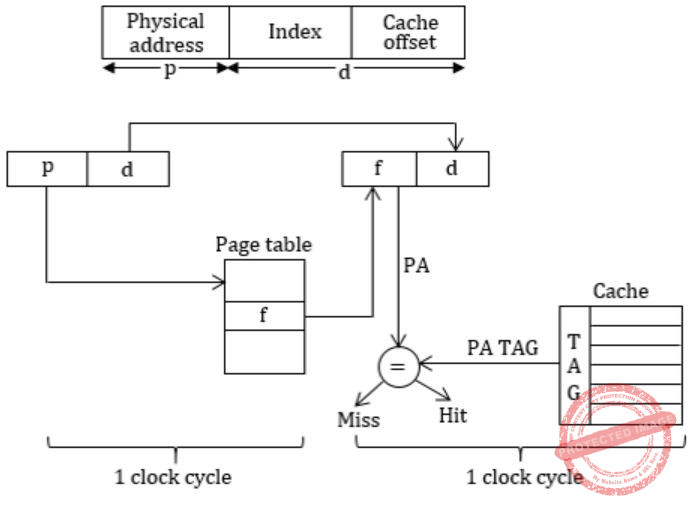Architecture of virtual indexed physically tagged (VIPT):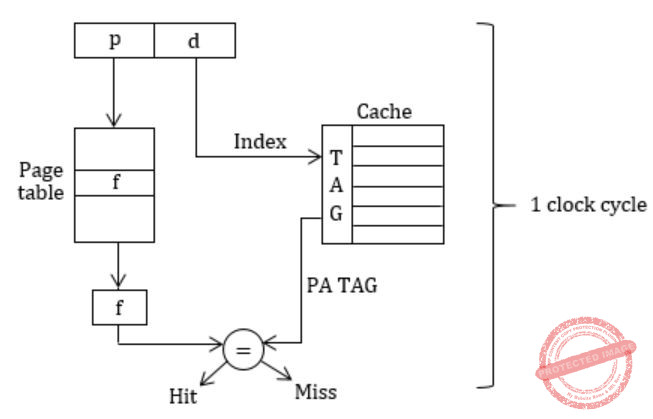VIPT cache and aliasing effect and synonym.
Synonym: Different virtual addresses mapped to same physical address (for data sharing).
So these synonyms should be in same set to avoid write-update problems.
In our problem VA = 46bitsWe are using 16bits for indexing into cache.
To have two synonym is same set we need to have same 16 bits index for PA & VA.
Assume that physical pages are colored and each set should have pages of same color so that any synonyms are in same set.
Since page size = 8KB ⇒ 13bits
These 13bits are not translated during VA→PA. So 13bits are same out of 16 Index bits, 13 are same we need to make 3bits (16-13) same now.
3bits can produce, 23 = 8 combinations which can be mapped on the different sets, so we need 8 different colors to color our pages. >br> In physically indexed cache indexing is done via physical address bits, but in virtual indexed cache, cache is indexed from (offset + set) bits. In physical Index cache indexing is done one to one (1 index maps to one page in one block of cache). In VIPT we have more/ extra bits, so mapping is not one-one. Hence these extra bits have to be taken care, such that if two virtual address refers to same page in cache block of different sets then they have to be assumed same i.e., we say of same color and put same color page in one set to avoid write update problems.
 Question 55

Relation R has eight attributes ABCDEFGH . Fields of R contain only atomic values.

F = {CH→G, A→BC, B→CFG, E→A, F→EG}, is a set of functional dependencies (FDs) so that F+ is exactly the set of FDs that hold for R.

How many candidate keys does the relation R have?

 A 3 B 4 C 5 D 6
Database-Management-System       Normalization
Question 55 Explanation:
 The attribute D is not part of any FD's. So D can be a candidate key or it may be part of the candidate key.
 Now D+ = {D}.
 Hence we have to add A,B,C,E,F,G,H to D and check which of them are Candidate keys of size 2.
BD+ = {ABCDEFGH}
ED+ = {ABCDEFGH}
FD+ = {ABCDEFGH}
 But CD+, GD+ and HD+ does not give all the attributes hence CD, GD and HD are not candidate keys.
 Hence no. of candidate keys are 4: AD, BD, ED, FD.
 Question 56

Relation R has eight attributes ABCDEFGH . Fields of R contain only atomic values.

F = {CH→G, A→BC, B→CFG, E→A, F→EG}, is a set of functional dependencies (FDs) so that F+ is exactly the set of FDs that hold for R.

The relation R is

 A in 1NF, but not in 2NF. B in 2NF, but not in 3NF. C in 3NF, but not in BCNF. D in BCNF.
Database-Management-System       Normalization
Question 56 Explanation:
 The attribute D is not part of any FD's. So D can be a candidate key or it may be part of the candidate key.
 Now D+ = {D}.
 Hence we have to add A,B,C,E,F,G,H to D and check which of them are Candidate keys of size 2.
BD+ = {ABCDEFGH}
ED+ = {ABCDEFGH}
FD+= {ABCDEFGH}
 But CD+, GD+ and HD+ does not give all the attributes hence CD, GD and HD are not candidate keys.
 Here Candidate keys are AD, BD, ED and FD.
 A → BC, B → CFH and F → EG etc are partial dependencies.
 So given relation is in 1NF, but not in 2NF.
 Question 57

Which one of the following options is the closest in meaning to the word given below?

` Nadir `
 A Highest B Lowest C Medium D Integration
Aptitude       Verbal
Question 57 Explanation:
Nadir = The lowest (or) most unsuccessful point in a situation.
 Question 58

Complete the sentence: Universalism is to particularism as diffuseness is to _________________

 A specificity B neutrality C generality D adaptation
Aptitude       Verbal
Question 58 Explanation:
Universalism = The belief that all human kind will eventually be saved.
Particularism = Exclusive attachment to one's own group, party (or) Nation.
Diffuseness = The spatial property of being spread out over a wide area (or) through a large volume.
Specificity = The quality of belonging (or) relating uniquely to a particular subject.
 Question 59

What will be the maximum sum of 44, 42, 40, ...... ?

 A 502 B 504 C 506 D 500
Aptitude       Numerical
Question 59 Explanation:
44, 42, 40, ......
44, 42, 40, ...... 0
2(22 + 21 + 20 + …. 1)
Sum(n) = (n(n+1))/2 = (22×23)/2 = 253
⇒ 2(253)
= 506
 Question 60

Were you a bird, you ______________ in the sky.

 A would fly B shall fly C should fly D shall have flown
Aptitude       Verbal
Question 60 Explanation:
Were you a bird, you would fly in the sky.
 Question 61

Choose the grammatically INCORRECT sentence:

 A He is of Asian origin. B They belonged to Africa. C She is an European. D They migrated from India to Australia.
Aptitude       Verbal
Question 61 Explanation:
"She is an European"
→ We can't use "an" infront of European. It can be pronounced as European. Here E is silent.
→ So, option C is the answer
 Question 62

Find the sum of the expression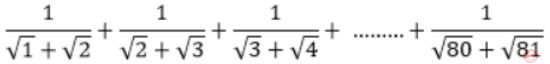A 7 B 8 C 9 D 10
Aptitude       Numerical
Question 62 Explanation:Multiply on numerator & denominator= -1+9
= 8
 Question 63

Out of all the 2-digit integers between 1 and 100, a 2-digit number has to be selected at random. What is the probability that the selected number is not divisible by 7?

 A 13/90 B 12/90 C 78/90 D 77/90
Aptitude       Numerical
Question 63 Explanation:
⇨ Total number of 2 digit numbers from 1 to 100 = 90
⇨ Find which can be divisible by 7 upto 100
14, 21, 28, 35, 42, 49, 56, 63, 70, 77, 84, 91, 98
Total = 13
Probability of divisible by 7 is = 13/90
Which can’t be divisible by 7 is
⇒ 1 – (probability of divisible by 7)
⇒ 1 – (13/90)
⇒ 77/90
 Question 64

After several defeats in wars, Robert Bruce went in exile and wanted to commit suicide. Just before committing suicide, he came across a spider attempting tirelessly to have its net. Time and again, the spider failed but that did not deter it to refrain from making attempts. Such attempts by the spider made Bruce curious. Thus, Bruce started observing the near-impossible goal of the spider to have the net. Ultimately, the spider succeeded in having its net despite several failures. Such act of the spider encouraged Bruce not to commit suicide. And then, Bruce went back again and won many a battle, and the rest is history. Which one of the following assertions is best supported by the above information?

 A Failure is the pillar of success. B Honesty is the best policy. C Life begins and ends with adventures. D No adversity justifies giving up hope.
Aptitude       Verbal
Question 64 Explanation:
Option A, B, C are not able to given correct sense about the information.
Option D is related to the given information.
 Question 65

A tourist covers half of his journey by train at 60 km/h, half of the remainder by bus at 30 km/h and the rest by cycle at 10 km/h. The average speed of the tourist in km/h during his entire journey is

 A 36 B 30 C 24 D 18
Aptitude       Numerical
Question 65 Explanation: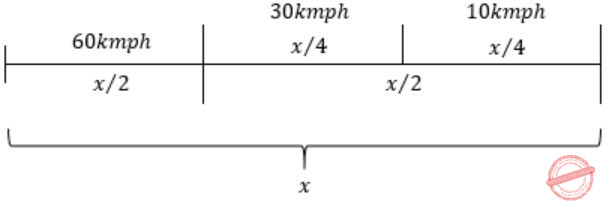Let ‘x’ be the total distance,
Speed = distance/ time
Total speed = (total distance)/(total time); Time = distance/speed
Time taken for train journey = (x⁄2)/60kmph = x/120
Time taken for bus journey = (x⁄4)/30kmph = x/120
Time taken for cycle journey = (x⁄4)/10kmph = x/40
Total time = x/120+x/120+x/40 = 5x/120
Total speed = (total distance)/(total time) = x/(5x⁄120) = (120×x)/5x = 24kmph
 Question 66

The current erection cost of a structure is Rs. 13,200. If the labour wages per day increase by 1/5 of the current wages and the working hours decrease by 1/24 of the current period, then the new cost of erection in Rs. is

 A 16,500 B 15,180 C 11,000 D 10,120
Aptitude       Numerical
Question 66 Explanation:
Current wages is increased by (1/5) ⇒ 1+1/5 ⇒ 6/5
Working hours decreased by (1/24) ⇒ 1-1/24 ⇒ 23/24
The new cost of erection = 13,200 × 6/5 × 23/24 = 15,180
There are 66 questions to complete.